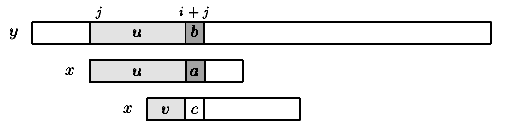Name
• String
• Substring
• Search
Edit
The design of the Morris-Pratt algorithm follows a tight analysis of the Brute Force algorithm, and especially on the way this latter wastes the information gathered during the scan of the text.

Let us look more closely at the brute force algorithm. It is possible to improve the length of the shifts and simultaneously remember some portions of the text that match the pattern. This saves comparisons between characters of the pattern and characters of the text and consequently increases the speed of the search.

Consider an attempt at a left position j on y, that is when the window is positioned on the text factor y[j .. j+m-1]. Assume that the first mismatch occurs between x[i] and y[i+j] with 0 < i < m. Then, x[0..i-1] = y[j .. i+j-1] = u and a = x[i]  y[i+j]=b.

When shifting, it is reasonable to expect that a prefix v of the pattern matches some suffix of the portion u of the text. The longest such prefix v is called the border of u (it occurs at both ends of u). This introduces the notation: let mpNext[i] be the length of the longest border of x[0 .. i-1] for 0 < i  m. Then, after a shift, the comparisons can resume between characters c=x[mpNext[i]] and y[i+j]=b without missing any occurrence of x in y, and avoiding a backtrack on the text (see figure 6.1). The value of mpNext is set to -1.Figure 6.1:  Shift in the Morris-Pratt algorithm (v border of u).

The table mpNext can be computed in O(m) space and time before the searching phase, applying the same searching algorithm to the pattern itself, as if x=y.

Then the searching phase can be done in O(m+n) time. The Morris-Pratt algorithm performs at most 2n-1 text character comparisons during the searching phase. The delay (maximal number of comparisons for a single text character) is bounded by m.
C